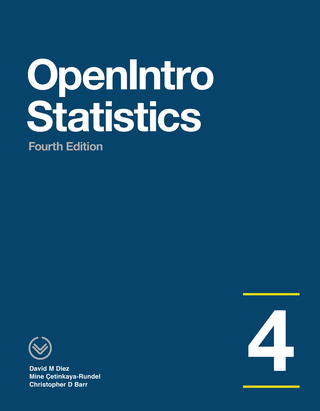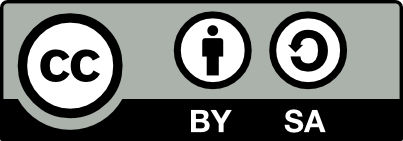It looks like you're using Internet Explorer 11 or older. This website works best with modern browsers such as the latest versions of Chrome, Firefox, Safari, and Edge. If you continue with this browser, you may see unexpected results.

OER: Open Educational Resources

This guide is designed for FSCJ faculty to discover learning objects and other open educational resources. Faculty will also find information on adding and uploading content to share with colleagues.

TextbooksOpenIntro Statistics by David Diez  (2019): OpenIntro.

OpenIntro Statistics offers a traditional introduction to statistics at the college level. This textbook is widely used at the college level and offers an exceptional and accessible introduction for students from community colleges to the Ivy League. The textbook has been thoroughly vetted with an estimated 20,000 students using it annually.Introductory Statistics follows scope and sequence requirements of a one-semester introduction to statistics course and is geared toward students majoring in fields other than math or engineering. The text assumes some knowledge of intermediate algebra and focuses on statistics application over theory. Introductory Statistics includes innovative practical applications that make the text relevant and accessible, as well as collaborative exercises, technology integration problems, and statistics labs.Bringing a fresh approach to intro statistics, ISRS introduces inference faster using randomization and simulation techniques.This is a free textbook teaching introductory statistics for undergraduates in Psychology. This textbook is part of a larger OER course package for teaching undergraduate statistics in Psychology, including this textbook, a lab manual, and a course website. All of the materials are free and copiable, with source code maintained in Github repositories.Learning Statistics with R covers the contents of an introductory statistics class, as typically taught to undergraduate psychology students, focusing on the use of the R statistical software. The book discusses how to get started in R as well as giving an introduction to data manipulation and writing scripts. From a statistical perspective, the book discusses descriptive statistics and graphing ﬁrst, followed by chapters on probability theory, sampling and estimation, and null hypothesis testing. After introducing the theory, the book covers the analysis of contingency tables, t-tests, ANOVAs and regression. Bayesian statistics are covered at the end of the book.Think Stats is an introduction to Probability and Statistics for Python programmers. Think Stats emphasizes simple techniques you can use to explore real data sets and answer interesting questions. The book presents a case study using data from the National Institutes of Health. Readers are encouraged to work on a project with real datasets.The book "Introductory Business Statistics" by Thomas K. Tiemann explores the basic ideas behind statistics, such as populations, samples, the difference between data and information, and most importantly sampling distributions. The author covers topics including descriptive statistics and frequency distributions, normal and t-distributions, hypothesis testing, t-tests, f-tests, analysis of variance, non-parametric tests, and regression basics. Using real-world examples throughout the text, the author hopes to help students understand how statistics works, not just how to "get the right number."